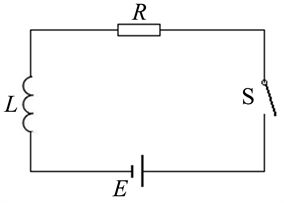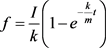﻿ 一类线性负反馈系统的数学描述

# 一类线性负反馈系统的数学描述Mathematical Description of a Class of Linear Negative Feedback Systems

Abstract: A linear negative feedback equation is constructed. The simplified mathematical description of the system’s own maintenance and adaptability to the environment of the system is made during the evolution of the closed system from one state to another.

1. 引言

2. 线性负反馈问题

2.1. 燃烧现象中的负反馈

$\frac{\text{d}\rho }{\text{d}t}=k\left({\rho }_{0}-\rho \right)$ ，其中k为比例系数。

$k{\rho }_{0}-k\rho =\frac{\text{d}\rho }{\text{d}t}$ (1)

$\int \frac{\text{d}\rho }{k{\rho }_{0}-k\rho }=\int \text{d}t$

$-\frac{1}{k}\mathrm{ln}\left(k{\rho }_{0}-k\rho \right)=t+{C}_{1}$

$\rho ={\rho }_{0}+C{e}^{-kt}$ (其中 $C=-\frac{{e}^{-k{C}_{1}}}{k}$ ) (2)

$\rho ={\rho }_{0}\left(1-{e}^{-kt}\right)$ (3)

2.2. 自由落体中的负反馈

$mg-kv=m\frac{\text{d}v}{\text{d}t}$ (4)

$v=\frac{mg}{k}\left(1-{e}^{-\frac{k}{m}t}\right)$ (5)

2.3. LR电路中的负反馈

$E-iR=L\frac{\text{d}i}{\text{d}t}$ (6)Figure 1. RL circuit

$i=\frac{E}{R}\left(1-{e}^{-\frac{R}{L}t}\right)$ (7)

3. 线性负反馈数学模型

3.1. 模型的建立

$I-kf=m\frac{\text{d}f}{\text{d}t}$ (8)(9)

3.2. 模型讨论及意义

3.2.1. 负反馈方程的性质

3.2.2. 负反馈方程的意义

1) 系统从一个平衡态到另一个平衡态发展的过程中，负反馈方程

$I-kf=m\frac{\text{d}f}{\text{d}t}$

2) 物体质量是物体惯性大小的量度，这一论断在负反馈方程的解

$f=\frac{I}{k}\left(1-{e}^{-\frac{k}{m}t}\right)$

LR电路中的电感系数L，和内禀常数m相比，同样起到延缓电流i增长的作用。

3) 在负反馈方程中，当诱导量I消失后，方程就和法拉第电磁感应定律方程形式相同，意义也相同。反馈系数k的符号，对应的是楞次定律的思想。当环境中反馈因子不存在时，方程就和牛顿第二定律形式相同。

4) 在封闭系统内，反馈系数k削减了诱导量I的作用，最终使f稳定在 $\frac{I}{k}$ 的大小。和内禀常数m相

5) 线性负反馈模型可以为更加复杂的非线性负反馈研究提供理论基础。

4. 结论

 钟永光, 贾晓菁, 钱颖. 系统动力学[M]. 第2版. 北京: 科学出版社, 2018: 103-107.

 胡斌. 电子线路轻松入门[Ｍ]. 第2版. 北京: 人民邮电出版社, 2006: 287.

 冯军, 谢嘉奎. 电子线路(线性部分) [M]. 第5版. 北京: 高等教育出版社, 2010: 292-318.

 同济大学数学教研室. 高等数学(下册) [M]. 第2版. 北京: 高等教育出版社, 1982: 315-316.

 赵凯华, 陈熙谋. 电磁学[M]. 第2版. 北京: 高等教育出版社, 2006: 209.

 包景东, 等. 从简化模型中悟出质点动力学的真谛[J]. 大学物理, 2017, 36(3): 1-4.

Top